# GRE Geometry | Three – Dimensional Figures

An object which has only height and length are called 2-dimensional figures while an abject which has height length and width are called 3-dimensional object.

Examples of 2-D figures:

Examples of 3-dimensional figures:

1. Cube: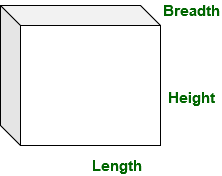In a cube length breadth and height are all equal.

`length = breadth = height `

let’s length, breadth and height as a.
It has 6 square face each with side length a.

```Volume of cube = a * a * a
Volume of cube = a3

Surface area of cube = 6 * area of one face
Surface area of cube = 6 * a2 ```
2. Cuboid: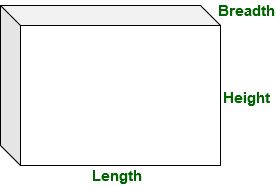In a cuboid length breadth and height are not equal.
It has 6 square face,

```Volume of cuboid = length * breadth * height

Surface area of cuboid = 6 * area of one face
Surface area of cuboid = length * breadth
+ length * height
+ length * height

Surface area of cuboid = 2(length * breadth
+ length * height ) ```
3. Sphere: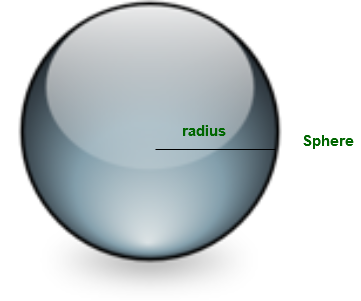A sphere has radius, volume and surface area:

```Volume of sphere = (4 / 3) * π * radius * radius * radius
Volume of sphere = (4 / 3) * π * r3

Surface area of sphere = 4 * &pi * radius * radius
Surface area of sphere = 4 * &pi * radius2

Surface area of sphere = 2 * &pi * radius * radius ```
4. Cylinder: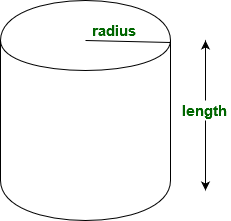A cylinder is a solid or hollow circular shape object with two circular base.

```Volume of cylinder = π * radius * radius * h
Curved surface area of cylinder = 2 * π * radius * h
Total surface area = 2 * π * radius * h + 2 * &pi * radius * radius ```
5. Cone: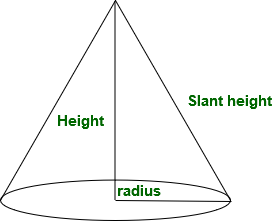A cylinder is a solid or hollow with one circular base and which tappers from base to a point.

```Volume of a cone  = (1 / 3)π * radius * radius * height

Curved surface area of a cone
= π * radius * slant height

Total surface area of cone
= π * radius * slant height
6. Pyramid: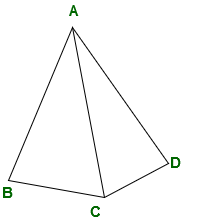Pyramid is 3-d object with polygon base, this polygon base is connected to an apex in pyramid.

```Volume of a pyramid
= (area of base * height) / 3 ```

Examples:

1. What will be the curved surface area of a cylinder having radius 5cm and height 10cm?
```Curved surface area of a cylinder
= 2 * π * r * height
= 2 * 22 / 7 * 5 * 10
= 314.285714286 cm2 ```
2. What will be the slant height of a cone which have curved surface area 990 cm2 and radius 5 cm?
```Curved surface area of a cone = π * radius * slant height
π * radius * slant height  = (22 / 7) * 5 * l
l = 63cm ```
3. What is the volume of a square base pyramid having base side 4 cm and height 6cm?
```Volume of a square
= area of base * height / 3
= 42 * 6 / 3
= 16 * 6 / 3
= 32 cm3 ```
4. What will be the volume of a sphere having radius 2.1cm
```Volume of a sphere
= 4 * π * radius3 / 3
= (4 / 3) * (22 / 7) * 2.13
= 38.808 cm3  ```
5. What will be the surface area of a cube having side lenghth breadth and height 8 cm?
```Surface area of a cube
= 6 * side2
= 6 * 82
= 6 * 64
= 384 cm2 ```

My Personal Notes arrow_drop_upCheck out this Author's contributed articles.

If you like GeeksforGeeks and would like to contribute, you can also write an article using contribute.geeksforgeeks.org or mail your article to contribute@geeksforgeeks.org. See your article appearing on the GeeksforGeeks main page and help other Geeks.

Please Improve this article if you find anything incorrect by clicking on the "Improve Article" button below.

Article Tags :

1

Please write to us at contribute@geeksforgeeks.org to report any issue with the above content.